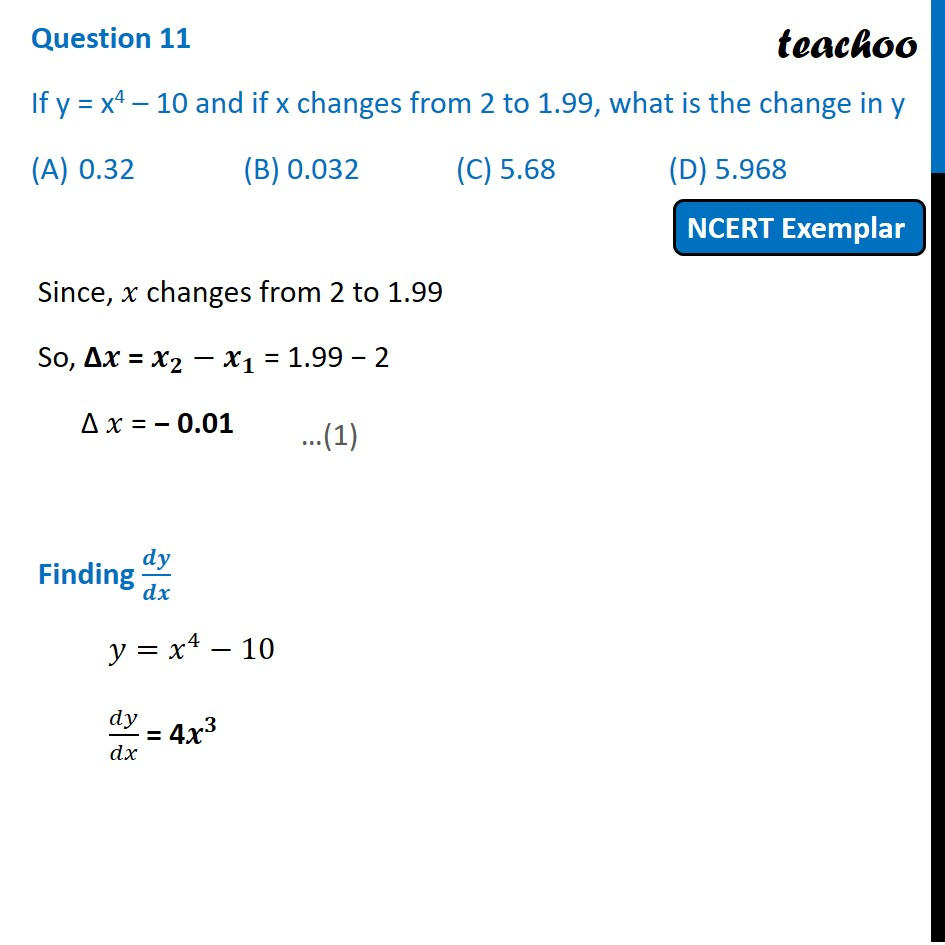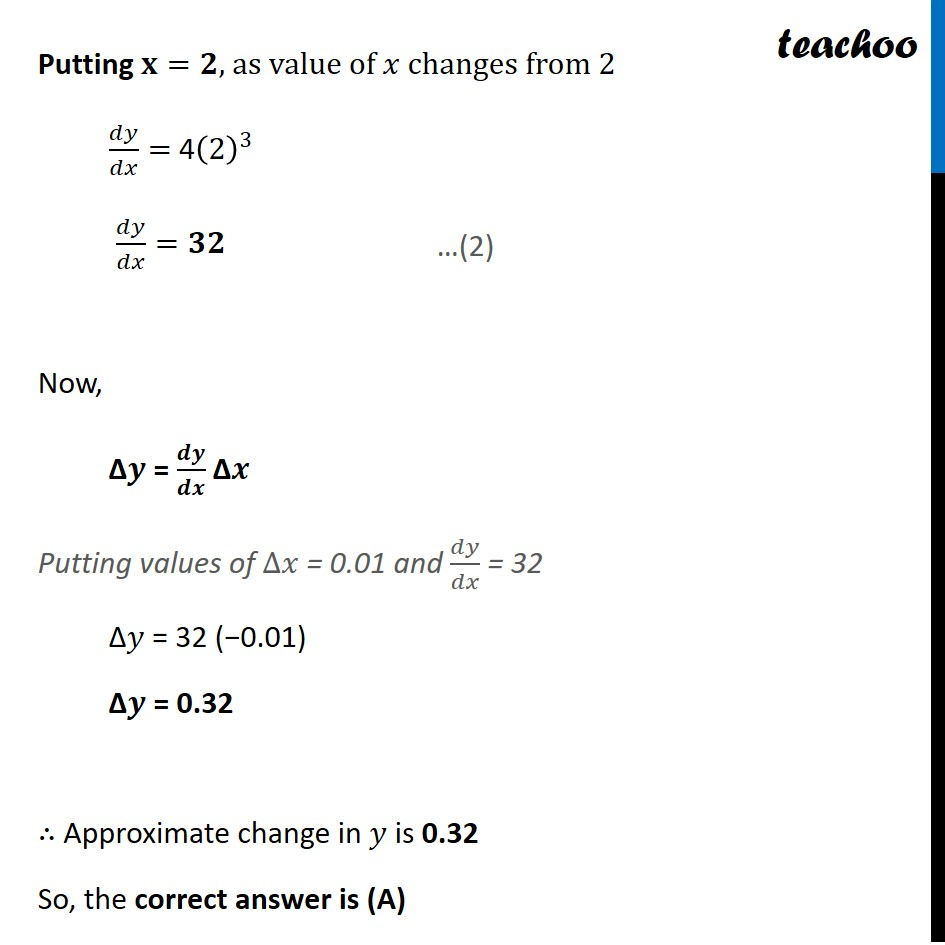NCERT Exemplar - MCQs

Chapter 6 Class 12 Application of Derivatives
Serial order wise

## (A)0.32   (B) 0.032   (C) 5.68   (D) 5.968Learn in your speed, with individual attention - Teachoo Maths 1-on-1 Class

### Transcript

Question 9 If y = x4 – 10 and if x changes from 2 to 1.99, what is the change in y 0.32 (B) 0.032 (C) 5.68 (D) 5.968 Since, 𝑥 changes from 2 to 1.99 So, ∆𝒙 = 𝒙_𝟐−𝒙_𝟏 = 1.99 − 2 ∆ 𝑥 = − 0.01 Finding 𝒅𝒚/𝒅𝒙 𝑦=𝑥^4−10 𝑑𝑦/𝑑𝑥 = 4𝒙^𝟑 Putting 𝐱=𝟐, as value of 𝑥 changes from 2 𝑑𝑦/𝑑𝑥= 4(2)^3 𝑑𝑦/𝑑𝑥=𝟑𝟐 Now, ∆𝒚 = 𝒅𝒚/𝒅𝒙 ∆𝒙 Putting values of ∆𝑥 = 0.01 and 𝑑𝑦/𝑑𝑥 = 32 ∆𝑦 = 32 (−0.01) ∆𝒚 = 0.32 ∴ Approximate change in 𝑦 is 0.32 So, the correct answer is (A)